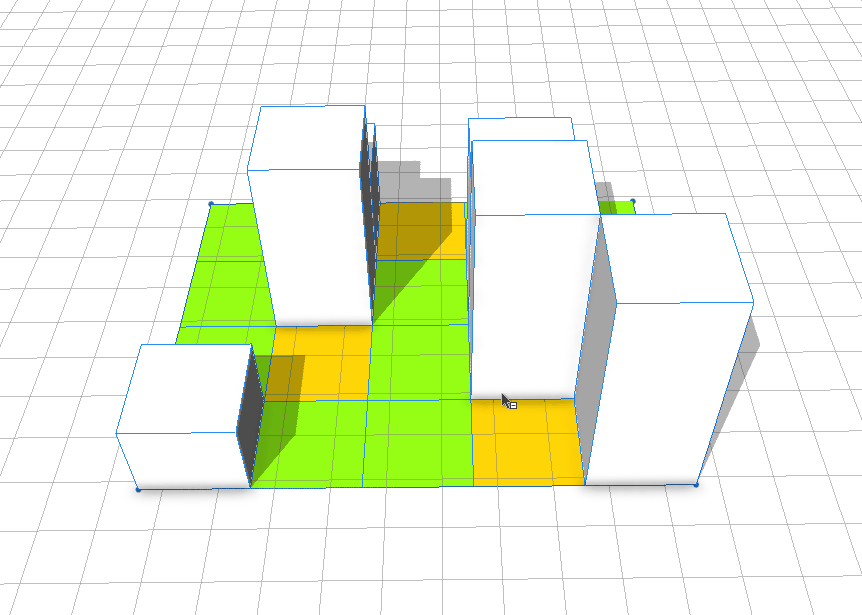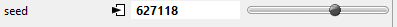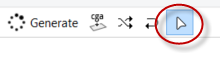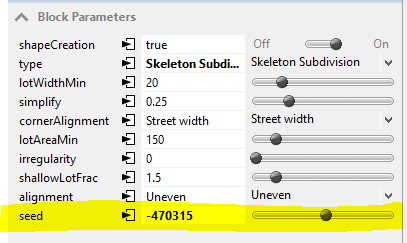# how to add seed function

753
5
11-23-2017 02:49 AMNew Contributor II

I have split a parcel into pieces and assigned a probability function between  building, greenspace and openspace.

I have also given rand height function for the building.

When I regenerate(ctrl+shift+g) it give me options like,But the issue is once I select the building footprint location I want to regenerate for random height , when I try to that it will change the building location also because of probability function.

I there a way, where I can apply seedian function just for height without changing the location of the building. something like thisThanks

Ab

Tags (3)
5 RepliesbyEsri Regular Contributor

If the initial shape is the large space which is divided up into the 4x5 grid, then trying to select one of the 20 individual cells is not possible in the normal selection mode.  The whole large shape will be selected, and then all 20 lots will be regenerated with different random numbers.  When updating the seed using Ctrl+Shift+G, the seed for the whole shape will be changed, so this will change your type assignment on the grid cells no matter what, which I think is not what you want.

To make changes to an individual cell, I would recommend using the local edits mode, which can be found on toolbar or accessible through the keyboard shortcut O.  You would have to create an attribute for the building height, put a handle on it, and then you can change the height of each individual building that you can select in local edits mode.``````@Handle(shape=Mass)
attr building_height = 10

GridCell -->
extrude(building_height)
Mass
‍‍‍‍‍‍``````

Here is a tutorial on local edits (only available starting with 2017.0).New Contributor II

Does @Handels works on 2015.2 advance version?

My height function is not defined in attribute, it from the GF_Height and UF_Height,

getHeight(row_ind, nRows, col_ind, nCols) = GfHeight + nFloors(row_ind, nRows, col_ind, nCols)*UfHeight

nFloors(row_ind, nRows, col_ind, nCols) =
case Variation_Mode == "None" || (nRows*nCols) < 2 : Floor_Max
case Variation_Mode == "Increasing" : (Floor_Min) + (rint((Floor_Max-Floor_Min)*((row_ind*nCols+col_ind)/((nRows*nCols)-1))))
case Variation_Mode == "Decreasing" : Floor_Min + rint((Floor_Max-Floor_Min)*(1-(row_ind*nCols+col_ind)/((nRows*nCols)-1)))
case Variation_Mode == "Alternating" : Floor_Min + rint((Floor_Max-Floor_Min)*((row_ind*nCols+col_ind)%2))
else

Lot -->

extrude(getHeight(row_ind, nRows, col_ind, nCols) )

I am looking for a seed function like in block division shape parameters. Is it possible to have such function in the code.byEsri Regular Contributor

Handles were first introduced in 2015.0, but they have been updated and extended since then.  Here is a tutorial on handles and some pages from the help doc:

Handles are only available on attributes.

The seed is not really a function but rather a number that belongs to the shape.  In cga code, you can get and set this number using the seedian shape attribute.New Contributor II

Thanks for the Tutorial, helped me a lot to understand more ways to write my code.

But I am not sure how to put a handle in my code since it is generated from

GFHeight + nFloors*UFHeight

Here is my complete code

Lot -->
split(x) { Width: Col(split.index, split.total)}*

Col(col_ind, nCols) -->
split(z) { Length: Cell(split.index, split.total, col_ind, nCols)}*

Cell(row_ind, nRows, col_ind, nCols) -->

extrude(getHeight(row_ind, nRows, col_ind, nCols) )

//////////////////////////

getHeight(row_ind, nRows, col_ind, nCols) = GfHeight + nFloors(row_ind, nRows, col_ind, nCols)*UfHeight

nFloors(row_ind, nRows, col_ind, nCols) =
case Variation_Mode == "None" || (nRows*nCols) < 2 : Floor_Max
case Variation_Mode == "Increasing" : (Floor_Min) + (rint((Floor_Max-Floor_Min)*((row_ind*nCols+col_ind)/((nRows*nCols)-1))))
case Variation_Mode == "Decreasing" : Floor_Min + rint((Floor_Max-Floor_Min)*(1-(row_ind*nCols+col_ind)/((nRows*nCols)-1)))
case Variation_Mode == "Alternating" : Floor_Min + rint((Floor_Max-Floor_Min)*((row_ind*nCols+col_ind)%2))
else : rint(rand(Floor_Min-0.49,Floor_Max+0.49))     //////////// Random Height Function

I can't put handle on both GFHeight and UFHeight in the attribute because both will be constant from the attribute.

I saw the seedian shape attribute in help link but didn't get it properly how to apply in the code. It will be great if you can provide some example on using "seedian shape attribute"

ThanksbyEsri Regular Contributor

Handles only work on attributes.  In order to use handles, you would have to redesign the code so that you have attributes for the quantities that you want to have handles for.

For example, you could put a handle on the attribute nFloors and then set the handle to use the shape which is the mass of the building which is extruded to the correct height.

As for the seedian, again, I don't think setting it will get you what you want, but maybe you see a way of getting it to work for you.  You can set it in cga code using set().

This sets the seedian to the value 123456, for example.

``set(seedian, 123456)‍‍``